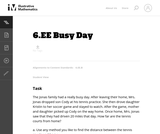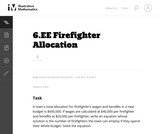Updating search results...

# 3 Results

View
Selected filters:
• MCCRS.Math.Content.6.EE.B.7Unrestricted Use
CC BY
Rating
3.0 stars

This task provides a good entry point for students into representing quantities in contexts with variables and expressions and building equations that reflect the relationships presented in the context.

Material Type:
Activity/Lab
Provider:
Illustrative Mathematics
Provider Set:
Illustrative Mathematics
Author:
Illustrative Mathematics
08/15/2012Unrestricted Use
CC BY
Rating
0.0 stars

In this task students are asked to write an equation to solve a real-world problem. There are two natural approaches to this task. In the first approach, students have to notice that even though there is one variable, namely the number of firefighters, it is used in two different places. In the other approach, students can find the total cost per firefighter and then write the equation.

Material Type:
Activity/Lab
Provider:
Illustrative Mathematics
Provider Set:
Illustrative Mathematics
Author:
Illustrative Mathematics
05/01/2012Only Sharing Permitted
CC BY-NC-ND
Rating
4.0 stars

This lesson unit is intended to help you assess how well students are able to: Perform arithmetic operations, including those involving whole-number exponents, recognizing and applying the conventional order of operations; Write and evaluate numerical expressions from diagrammatic representations and be able to identify equivalent expressions; apply the distributive and commutative properties appropriately; and use the method for finding areas of compound rectangles.

Material Type:
Assessment
Lesson Plan
Provider:
Shell Center for Mathematical Education
Provider Set:
Mathematics Assessment Project (MAP)